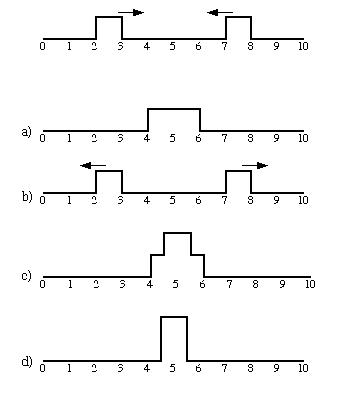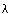TUTORIAL QUESTIONS 45-55

Questions:
45) Rectangular Pulses.
50) Wave Terminology.
55) Physical phenomena.

45.  Consider the two "rectangular" pulses below moving in opposite directions on a string at a propagation speed (i.e., wave velocity) of 1/2 ft/s.  What does the string look like 5 seconds afterward?a) b) c) d)

Fundamentals of Sound reference: Secs. 1-C, 1-K.

50.  For sinusoidal waves, match the term with its appropriate definition:

a)      Wavelength  (Symbol)
b)      Cycle
c)      Period  (Symbol T)
d)      Frequency  (Symbol f)
e)      Amplitude  (Symbol A)
f)      Displacement

1)      Time it takes a point in the medium to make one complete round trip.
(a),    (b),   (c)(d)(e)(f).
2)      The number of complete round trips that a point in the medium makes
in one second.
(a),    (b),   (c)(d)(e)(f).
3)      Maximum distance that a point in the medium moves away from
equilibrium during a    cycle.
(a),    (b),   (c)(d)(e)(f).
4)      Distance between two adjacent crests in the wave.
(a),    (b),   (c)(d)(e)(f).
5)      One complete round trip of a point in the medium.
(a),    (b),   (c)(d)(e)(f).
6)      Distance that a point in the medium has moved from equilibrium.
(a),    (b),   (c)(d)(e)(f).

Fundamentals of Sound reference: Sec. 1-G.

55.  Can the concept of a wave be used to describe the following physical phenomena?

i) a swinging pendulum
ii) a mass oscillating at the end of a spring

a) i only
b) ii only
c) both i and ii
d) neither i nor ii

Fundamentals of Sound reference: Sec. 1-E.